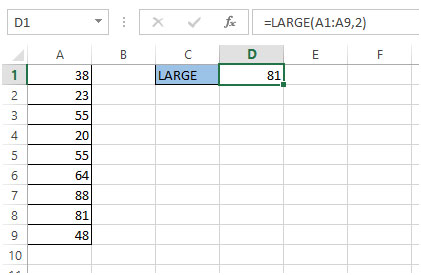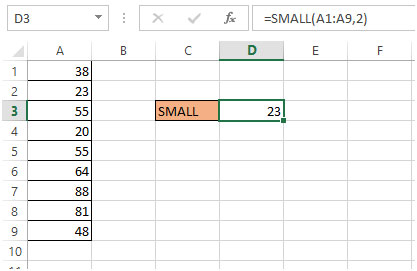# Returning the Nth Largest / Smallest Values in a Range in Microsoft Excel 2010

If we have a list and we want to find the nth largest or smallest number then we can use the LARGE and SMALL functions.

LARGE function:

Syntax: =LARGE(array,k)

Example:

We have a list of numbers in A1:A9. We need to get the 2nd largest number from this list.

1. Go to Cell D1
2. Type the LARGE formula
3. =LARGE(A1:A9,2)
4. Press Enter on your keyboard
5. From the list 81 is the 2nd largest number
6. We get the result as 81 in cell D1.SMALL function:

Syntax: =SMALL(array,k)

Example:

We have a list of numbers in A1:A9. We need to get the 2nd largest number from this list.

1. Go to Cell D3
2. Type the SMALL formula
3. =SMALL(A1:A9,2)
4. Press Enter on your keyboard
5. From the list 23 is the 2nd smallest number
6. We get the result as 23 in cell D31.If you want the second highest number you can use

=LARGE(A1:A9,2)

although that doesn't account for duplicates so you could get the same result as the Max

If you want the largest number that is smaller than the maximum number you can use this version

=LARGE(A1:A9,COUNTIF(A1:A9,MAX(A1:A9))+1)

•Hi Sathish,
How can i get the 3rd largest
=LARGE(A1:A9,COUNTIF(A1:A9,MAX(A1:A9))+2) seems not working.

2.If you want the largest number that is smaller than the maximum number you can use this version

=LARGE(A1:A9,COUNTIF(A1:A9,MAX(A1:A9))+1)

3.how to find 2nd largest no by index+macth
plz share wth me ths answer…

4.how to find send largest no by index+macth
plz share wth me ths answer...

5.Wonderful tutorial. Very clear explanation. Thank you.

6.How would you eliminate the values if there are duplicates? For example, in your sample list, if the value 88 is repeated and you use the same formula, it will return 88 instead of 81. I want the 2nd highest value regardless of any duplicate values.

•Did you ever find an answer for this issue of duplicates?

•=LARGE(F3:F28,COUNTIF(F3:F28,MAX(F3:F28))+1)

•Trying to find the next smallest but there are multiple duplicates. Any ideas?

7.4 5 3 1 7 5 3 8
sort it using selective sort and k=2

•=+LARGE({4,5,3,1,7,5,3,8},2)

Terms and Conditions of use

The applications/code on this site are distributed as is and without warranties or liability. In no event shall the owner of the copyrights, or the authors of the applications/code be liable for any loss of profit, any problems or any damage resulting from the use or evaluation of the applications/code.